## Example Questions

### Example Question #1 : How To Divide Decimals

If the cost of 20 equally priced pencils cost $5.80, then at the same rate what is the greatest number of pencils you can purchase for$50?

172

173

150

172.41

200

172

Explanation:

Remeber for this problem that you cannot spend more than $50. First find the price of each pencil by 5.80/20 = 29 cents. And then use this to do 50/0.29 = 172.41. So the greatest number of pencils you can purchase for$50 is 172 pencils.

### Example Question #9 : Decimal Operations

Charlie has charges on his credit card of $63.70,$37.32, and $81.78. If he pays down half his bill at once, and then pays the rest in four equal monthly installments, how much is each monthly installment? Possible Answers: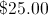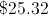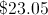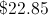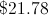Correct answer:Explanation: Adding all the charges together and dividing by 2 yields$91.40. Dividing this by 4 yields a monthly installment of \$22.85.

### Example Question #1 : How To Divide Decimals

Simplify: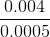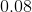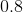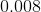Explanation:

Multiply both the numerator and denominator by some power of 10 to get rid of the decimals; in this case, multiply both by 10,000.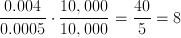### Example Question #1 : How To Order Decimals From Least To Greatest Or From Greatest To Least

Order the following decimals in order from least to greatest:

2.1, 6.9, 4.8, 5.2, 8.5

2.1, 4.8, 5.2, 6.9, 8.5

6.9, 4.8, 8.5, 5.2, 2.1

8.5, 6.9, 5.2, 4.8, 2.1

2.1, 5.2, 8.5, 4.8, 6.9

2.1, 4.8, 5.2, 6.9, 8.5

Explanation:

Look at the ones place first, ignoring the decimal. The order from greatest to least is 2, 4, 5, 6, and 8. Because none of the numbers in the ones place are the same, it doesn't matter what the decimal will be on these numbers- the order will remain the same. The order is 2.1, 4.8, 5.2, 6.9, 8.5.

### Example Question #2 : How To Order Decimals From Least To Greatest Or From Greatest To Least

In the number 5,783.2935, what is the digit in the hundredths place?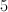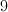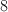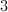Explanation:

The digit in the hundredths place is the one two decimal places to the right of the decimal. That digit is 9. Do not confuse the hundredths with the hundreds place, which is three spaces to the left of the decimal.

### Example Question #3 : How To Order Decimals From Least To Greatest Or From Greatest To Least

Which of these decimals are the greatest?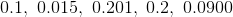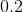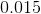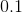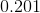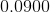Explanation:

look at the first decimal place; 0.2 and 0.201 are the largest.

0.2 = 0.200 and 0.201 > 0.200

0.201 is the greatest given decimal.

### Example Question #1 : How To Order Decimals From Least To Greatest Or From Greatest To Least

Which of the following is the greatest in value?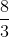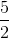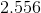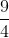Explanation:

Turn the fractions into decimals to easily compare them. You can do this quickly on your calculator.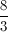is approximately 2.667, so this is the largest number.

### Example Question #1 : How To Order Decimals From Least To Greatest Or From Greatest To Least

Which of these decimals has the least value?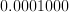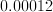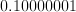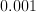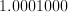Explanation:

Trailing zeros at the end of a decimal do not increase or decrease the value of a given decimal. However, zeros that appear after the decimal point and precede a non-zero number decrease the value of the number.

### Example Question #1 : How To Order Decimals From Least To Greatest Or From Greatest To Least

Put the following in order of least to greatest: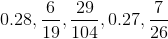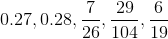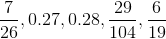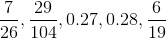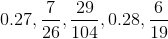Explanation:

Begin by converting each fraction into a decimal: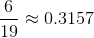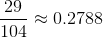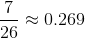Now we can put the decimals in order:

0.269, 0.27, 0.2788, 0.28, 0.3157

or: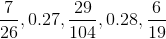### Example Question #1 : How To Order Decimals From Least To Greatest Or From Greatest To Least

Order the decimals from least to greatest.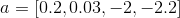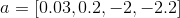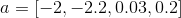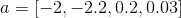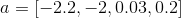Explanation:

The higher negative numbers will be the least numbers.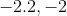The smaller of the two positive decimals is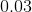.
The answer is: